Next: Jupiter Up: Planetary Latitudes Previous: Determination of Ecliptic Latitude

# Mars

The ecliptic latitude of Mars can be determined with the aid of Tables 46, 67, and 68. Table 46 allows the mean argument of latitude,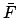, of Mars to be calculated as a function of time. Next, Table 67 permits the deferential latitude,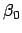, to be determined as a function of the true argument of latitude,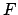. Finally, Table 68 allows the quantities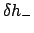,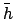, and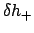to be calculated as functions of the epicyclic anomaly,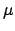.

The procedure for using the tables is as follows:

1. Determine the fractional Julian day number,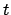, corresponding to the date and time at which the ecliptic latitude is to be calculated with the aid of Tables 27-29. Form, where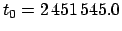is the epoch.
2. Calculate the planetary equation of center,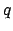, ecliptic anomaly,, and interpolation parameters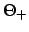and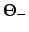using the procedure set out in Cha. 8.
3. Enter Table 46 with the digit for each power of 10 in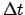and take out the corresponding values of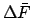. Ifis negative then the corresponding values are also negative. The value of the mean argument of latitude,, is the sum of all thevalues plus the value ofat the epoch.
4. Form the true argument of latitude,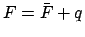. Add as many multiples oftoas is required to make it fall in the rangeto. Roundto the nearest degree.
5. Enter Table 67 with the value ofand take out the corresponding value of the deferential latitude,. It is necessary to interpolate ifis odd.
6. Enter Table 68 with the value ofand take out the corresponding values of,, and. If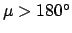then it is necessary to make use of the identities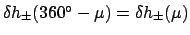and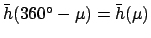.
7. Form the epicyclic latitude correction factor,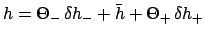.
8. The ecliptic latitude,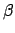, is the product of the deferential latitude,, and the epicyclic latitude correction factor,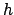. The decimal fraction can be converted into arc minutes using Table 31. Round to the nearest arc minute.
One example of this procedure is given below.

Example: May 5, 2005 CE, 00:00 UT:

From Cha. 8,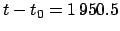JD,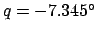,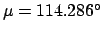,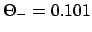, and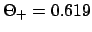. Making use of Table 46, we find:(JD)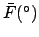+1000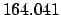+900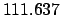+50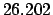+.5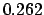Epoch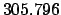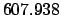Modulus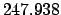Thus,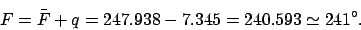It follows from Table 67 that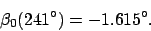Since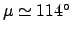, Table 68 yields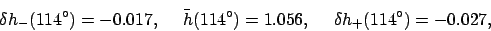so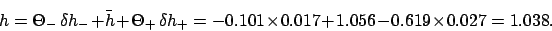Finally,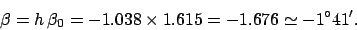Thus, the ecliptic latitude of Mars at 00:00 UT on May 5, 2005 CE was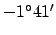.Next: Jupiter Up: Planetary Latitudes Previous: Determination of Ecliptic Latitude
Richard Fitzpatrick 2010-07-21